# QuizQ

An isosceles triangle has two sides of length 7 km and 39 km. How long is a third side?

Correct result:

x =  39 km

#### Solution: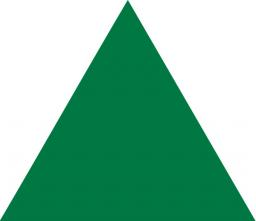We would be pleased if you find an error in the word problem, spelling mistakes, or inaccuracies and send it to us. Thank you!Tips to related online calculators

#### You need to know the following knowledge to solve this word math problem:

We encourage you to watch this tutorial video on this math problem:

## Next similar math problems:

• The perimeter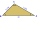The triangle has one side 5 cm long and the another 11 cm long. What can be the smallest and what is the largest perimeter?
• 3-bracket 3Two angles in a triangle are 90° and 60°. Has triangle at least two equal sides?
• Isosceles triangleThe circumference of the isosceles triangle is 32.5 dm. Base length is 153 cm. How long is the leg of this triangle?
• The cyclistThe cyclist drove from A to B, 60 km away. The first half drove at 30km/h, the second at 20km/h. How long did it take for him?
• Triangle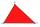For how many integer values of x can 16, 15 and x be the lengths of the sides of triangle?
• TrianglesEquilateral triangle with side 40 cm has the same perimeter as an isosceles triangle with arm of 45 cm. Calculate the base x of an isosceles triangle.
• MidpointsTriangle whose sides are midpoints of sides of triangle ABC has a perimeter 45. How long is perimeter of triangle ABC?
• Long drive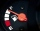Calculate how much it costs to drive 150,000 km with an average consumption of 7 liters per 100 km and a fuel price of 1.2 € / l.
• Cuboid aquariumCuboid 25 times 30 cm. How long is third side if cuboid contains 30 liters of water?
• SimilarityAre two right triangles similar to each other if the first one has an acute angle 70°, and the second one has an acute angle 20°?
• Chase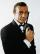Paul travel at 60 km/h. After 18 km ride found that he forgot an important book. His brother Thomas behind him carrying it. How fast must Thomas go to catch up with Paul in 31 minutes?The railroad runs parallel to the railway. The train travels at 36 km/h on track. In the opposite direction, the motorcycle rides at 90 km/h. The train passes in 3 seconds. How long is a train?I drive at an average speed of 70km/h, I drive 10 thousand km. How long did it take me?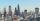The distance between two cities is actually 30 km and the map is 6 cm. What is the scale of the map?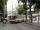Trolleybus line No. 206 measured 24 km. If the trolleybus goes faster by 5 km/h, the way there and back would is shorter by 33 minutes. Calculate the trolleybus speed and how much time it takes a return trip.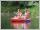Students of canoeists trip traveled in three days 102 km. Second day traveled 15% more than first day and at the third day 9 km over the second day. How many kilometers traveled each day?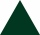The perimeter of an equilateral triangle is 33cm. How long is each side?